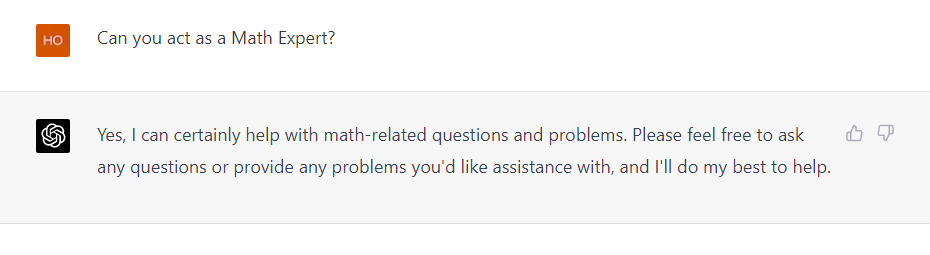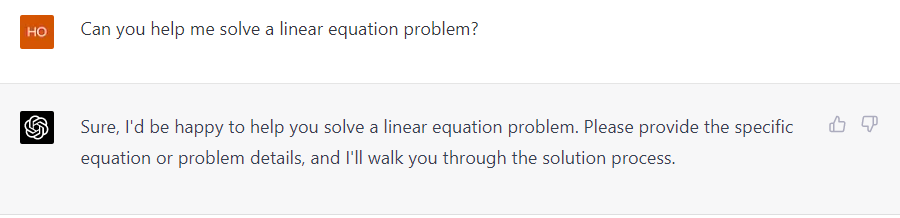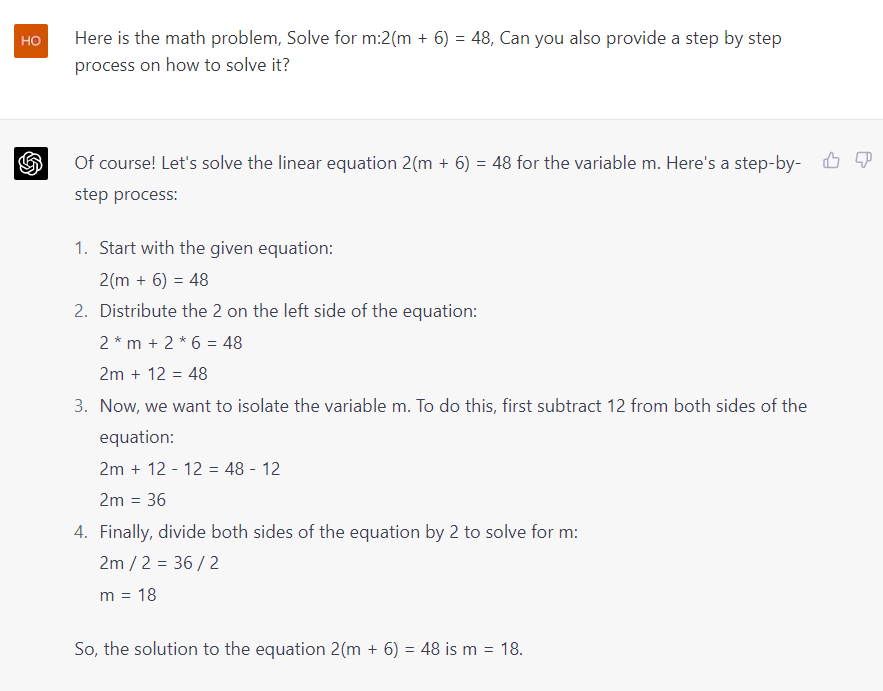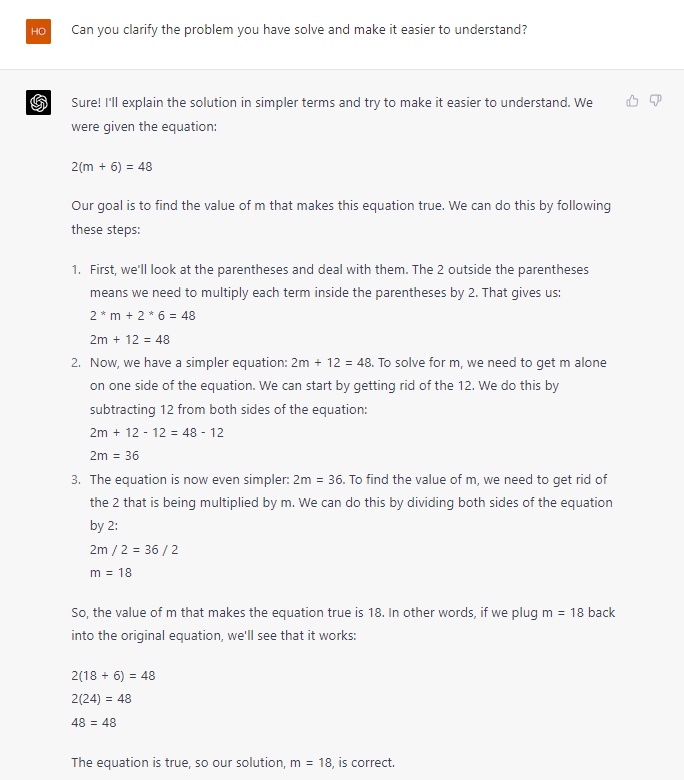How to AI

# How to Use ChatGPT for Math Problems – A Comprehensive GuideMathematics is a crucial subject that is taught in almost every school around the world. However, only some are comfortable with the subject, and many students need help with mathematical concepts. To overcome this challenge, we have ChatGPT, an AI-powered tool that can help solve complex mathematical problems. In this article, we will help you to let you know how to use ChatGPT for math and provide a step-by-step guide on how to make the most out of this tool.

## What is ChatGPT, and How Does It Work?

ChatGPT is an AI-powered tool that uses machine learning algorithms to understand human language and provide relevant responses. It is a language model that instructs on a massive amount of data to do it capable of understanding and processing a vast range of topics. The model uses a technique method called “transformer architecture,” which enables it to understand the context of the problem and generate accurate responses. Learning how to use ChatGPT will benefit you in many things including solving math problems, check how:

## How to Use ChatGPT for Maths: A Step-by-Step Guide

### 1. Make an Account

Create an account on the ChatGPT website to post the math problem.### 2. Set Your ChatGPT as a Math Expert

You can set a command to ChatGPT and ask it to act as a Math Expert. By doing this, it will allow ChatGPT to analyze your question easier.

Prompt: Can you act as a Math Expert?Ask the mathematical problem that you are having in simple terms.

Prompt: Can you help me solve a linear equation problem?### 4. Be Specific

ChatGPT will provide step-by-step guidance on addressing the issue based on the question.

Prompt: Here is the math problem, Solve for m:2(m+6) = 48, Can you also provide a step by step process on how to solve it?When you get stuck or need help comprehending a specific subject, ask questions, usually for further clarity. This will help you avoid becoming frustrated.

Prompt: Can you clarify the problem you have solve and make it easier to understand?## Tricks to Make the Most Out of ChatGPT for Maths

• Break down complex problems: If you have a difficult situation, try breaking it down into smaller parts and asking about each piece separately.

• Rephrase your question: If you are not getting a satisfactory response, try rephrasing your question using different words.

• Use the Math Solver feature: If you are not getting the desired response, consider using the “Math Solver” feature, which can provide step-by-step solutions to complex problems.

## FAQs

• ### Is ChatGPT free to use?

Yes, ChatGPT is entirely free to use. You do not need to pay fees or subscriptions to access this tool.

• ### Can ChatGPT solve all types of mathematical problems?

While ChatGPT can solve a wide range of mathematical problems, there may be some problems it cannot. If you cannot solve your situation, consider consulting a qualified mathematician.

• ### Can ChatGPT provide step-by-step solutions to problems?

Yes, ChatGPT has a “Math Solver” feature that can provide step-by-step solutions to complex problems.

## Conclusion

ChatGPT is an excellent tool for anyone who needs help with mathematics. Its advanced machine-learning algorithms can quickly accurately analyse and solve complex mathematical problems rapidly and accurately. By following the tips and tricks provided in## 5 Best Free AI Travel Planner: Plan Your Trip In Easiest Way

Let’s be honest crafting the perfect travel itinerary can be...## How To Use Looka AI: Your AI Logo Maker Guide

A well-designed logo is crucial for establishing a brand identity...